## Solution to 1986 Problem 6

 The only forces on the particle are gravity and the normal force. The normal force acts in a direction orthogonal to the tangential direction. Therefore, it does not contribute to the acceleration. So, we need only find the component of the gravitational acceleration in the tangential direction. The gravitational acceleration is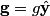\begin{align*}\mathbf{g} = g\hat{\mathbf{y}}\end{align*}The derivative of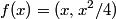$f(x) = (x,x^2/4)$ with respect to$x$ gives a tangent vector: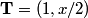\begin{align*}\mathbf{T} = (1,x/2)\end{align*}So, the UNIT tangent vector is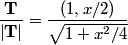\begin{align*}\frac{\mathbf{T}}{\left| \mathbf{T} \right|} = \frac{(1,x/2)}{\sqrt{1+ x^2/4}}\end{align*}Therefore, the answer is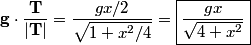\begin{align*}\mathbf{g} \cdot \frac{\mathbf{T}}{\left| \mathbf{T} \right|} = \frac{g x/2}{\sqrt{1+ x^2/4}} = \boxed{\frac{gx...Therefore, answer (D) is correct.